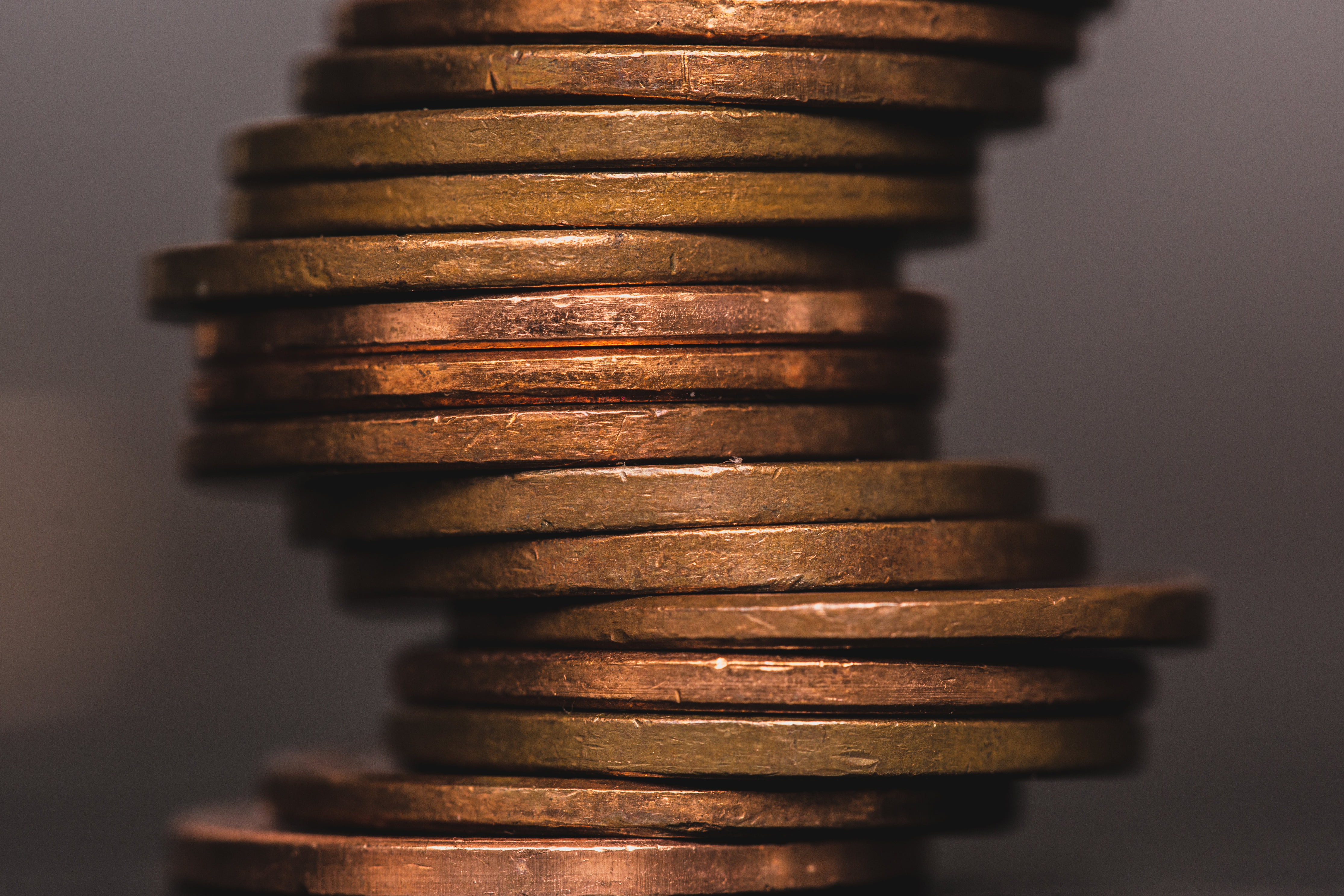<- Back

# Depreciation

Depreciation is a financial concept that refers to the decrease in value of an asset over time due to wear and tear, obsolescence, or other factors## What is depreciation?

Depreciation is a financial concept that refers to the decrease in value of an asset over time due to wear and tear, obsolescence, or other factors. It is an important concept in accounting and financial analysis. Understanding depreciation helps in assessing the true cost of using an asset and determining its remaining value.

Different methods, such as straight-line depreciation and declining balance depreciation, can be used to calculate and record depreciation. By accounting for depreciation, individuals and businesses can accurately reflect the value of their assets and make informed financial decisions.

#### Key takeaways

- Depreciation is the decrease in value of an asset over time.
- It is important for assessing the true cost of using an asset.
- Various methods can be used to calculate and record depreciation.## Understanding depreciation

Depreciation is a term used to describe the decline in value of an asset over its useful life. Here's how it works:

1. Asset value: When you buy an asset, such as a car or a computer, it has a certain value. However, over time, the asset's value decreases due to factors like regular wear and tear, technological advancements, or changes in market demand.

2. Useful life: Each asset has a useful life, which is the estimated period during which it is expected to provide value. For example, a computer might have a useful life of five years before it becomes outdated and needs to be replaced.

3. Depreciation methods: There are different methods to calculate and record depreciation. The most common method is straight-line depreciation, where the asset's value decreases by an equal amount each year. Another method is declining balance depreciation, where a higher percentage of the asset's value is depreciated in the earlier years.

## Real-world example of depreciation

Let's say you buy a car for £20,000. The useful life of the car is estimated to be ten years. Using straight-line depreciation, you would divide the car's value by its useful life to calculate the annual depreciation expense. In this case, it would be £2,000 per year (£20,000 ÷ 10).

By accounting for depreciation, you can better understand the actual cost of owning and using the car over time. It also helps in determining the car's remaining value and deciding when it might be more cost-effective to sell or replace it.

## Final thoughts on depreciation

Depreciation is the decrease in value of an asset over time. It is important for assessing the true cost of using an asset and understanding its remaining value. Different methods can be used to calculate and record depreciation, such as straight-line depreciation and declining balance depreciation. By considering depreciation, individuals and businesses can accurately reflect the value of their assets and make informed financial decisions.

We are committed to educating and empowering women to take control of their finances and to live life on their own terms.

CONTACT